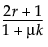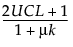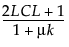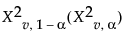P(X r) ~ P(X2v <)
Based on this approximation, approximate upper and lower control limits can be determined. For a nominal level α Type 1 error probability in one direction, an approximate upper control limit is a limit UCL such that:
P(X > UCL) = 1 - P(X2v <) = α
P(X < LCL) = 1 - P(X2v >) = αis the upper (lower) percentile of the chi-square distribution with v = 2μ/(1+μk) degrees of freedom. Negative lower control limits can be set to zero.

Help created on 9/19/2017